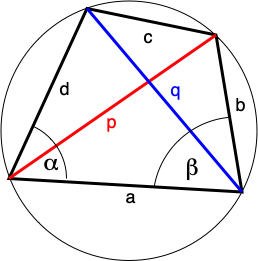# A generalization of the law of tangents

The law of tangents is a statement about the relationship between the tangents of two angles of a triangle and the lengths of the opposing sides.

Let $$a$$, $$b$$, and $$c$$ be the lengths of the three sides of a triangle, and $$\alpha$$, $$\beta$$ and $$\gamma$$ be the angles opposite those three respective sides. The law of tangents states that

$$\frac{\tan\frac12(\alpha-\beta)}{\tan\frac12(\alpha+\beta)}=\frac{a-b}{a+b}.\tag{1}\label{1}$$

The law of tangents can be used in any case where two sides and the included angle, or two angles and a side, are known.

Although Viète gave us the modern version of the law of tangents, it was Fincke who stated the law of tangents for the first time and also demonstrated its application by solving a triangle when two sides and the included angle are given (see Wu - The Story of Mollweide and Some Trigonometric Identities).

A proof of the law of tangents is provided by Wikipedia (see here).

Generalization. Let $$a$$, $$b$$, $$c$$ and $$d$$ be the sides of a cyclic convex quadrilateral. Let $$\angle{DAB}=\alpha$$ and $$\angle{ABC}=\beta$$, then the following identity holds

$$\frac{\tan\frac12(\alpha-\beta)}{\tan\frac12(\alpha+\beta)}=\frac{(a-c)(b-d)}{(a+c)(b+d)}.\tag{2}\label{2}$$

Proof. Using the sum-to-product formulas we can rewrite the left-hand side of $$(2)$$ as follows

$$\frac{\tan\frac12(\alpha-\beta)}{\tan\frac12(\alpha+\beta)}=\frac{\sin\frac12(\alpha-\beta)\cos\frac12(\alpha+\beta)}{\cos\frac12(\alpha-\beta)\sin\frac12(\alpha+\beta)}=\frac{\sin{\alpha}-\sin{\beta}}{\sin{\alpha}+\sin{\beta}}.$$

The area of a cyclic quadrilateral can be expressed as $$\Delta=\frac12(ad+bc)\sin{\alpha}$$ (see $$(12)$$ at Killing three birds with one stone) and similarly for the other angles. Then substituting, simplifying and factorizing we have

\begin{align*}\frac{\tan\frac12(\alpha-\beta)}{\tan\frac12(\alpha+\beta)}&=\frac{\frac{2\Delta}{ad+bc}-\frac{2\Delta}{ab+cd}}{\frac{2\Delta}{ad+bc}+\frac{2\Delta}{ab+cd}}=\frac{ab-ad+cd-bc}{ab+ad+cd+bc}=\frac{(a-c)(b-d)}{(a+c)(b+d)}\end{align*}.

$$\square$$

The formula \eqref{2} reduces to the law of tangents for a triangle when $$c=0$$.

A related result can be found at A generalization of Mollweide's Formula (rather Newton's).

Crossposted at MathSE.

Question: Is this generalization known?

A historical correction: Fincke was not the first to publish the law of tangents. It was actually Ibn Muadh who first described it in the 11th century. See the discussion at HSMSE.

• @CarloBeenakker this is useful to mention, but also including the posting date. The MathSE question was posted on March 16, 2022.
– YCor
Mar 29, 2022 at 12:37
• How do the sides $a,b,c,d$ match up to the vertices $A,B,C,D$? In a quadrilateral, there is no unambiguous concept of the side opposite a given angle. Mar 31, 2022 at 4:07
• @GerryMyerson I have asked myself the same question. Suppose we have a quadrilateral $ABCD$. The only sides that could be considered opposite to vertex $A$ are $BC$ or $CD$. Mar 31, 2022 at 12:54
• @YCor: Still, one is expected to put links on both questions and make it clear for the readers that there is another copy out there. Apr 1, 2022 at 7:40
• Hi @EmmanuelJoséGarcía , you might want to link to the MSE post in the body of your question; discussions in comments are often temporary, once their purpose has been served they can go; questions and answers are the place to store permanent info. Apr 1, 2022 at 11:58

To place the formula of the OP into context, it is helpful to note the identity (from Josefsson - More characterizations of cyclic quadrilaterals)

$$\frac{q-p}{q+p}=\frac{(a-c)(b-d)}{(a+c)(b+d)}.\tag{*}\label{star}$$ Here $$q$$ and $$p$$ are the lengths of the blue and red diagonals, with opposite angles $$\alpha$$ and $$\beta$$.The quadrilateral tangent formula can thus be rewritten in a form that looks more like the triangle tangent formula, $$\frac{\tan\frac12(\alpha-\beta)}{\tan\frac12(\alpha+\beta)}=\frac{q-p}{q+p}.$$

Q: Is it obvious that the law of tangents applies to the blue and red diagonals?

The formula \eqref{star} is equivalent (upon rearrangement of the terms) to what is known as Ptolemy’s second theorem $$\frac{p}{q}=\frac{ad+bc}{ab+cd}.$$
• In a private communication via gmail, Martin Josefsson commented: "As far as I can recall, I have not seen any of them, at least not in modern books or papers, and even if some of them where to be found in an old text, they are at least not well known, and deserve to be wider known." Apr 4, 2022 at 11:26
• I guess that settles it, if anyone should know it's Martin. Apr 4, 2022 at 11:35
• Yes, because at the core of the argument is $p=d \sin(\beta)$ where $d$ is the diameter of the circle. Apr 7, 2022 at 1:50